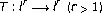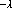# On a Hilbert-Type Operator with a Symmetric Homogeneous Kernel of1-Order and Applications

## Abstract

Some character of the symmetric homogenous kernel of1-order in Hilbert-type operatoris obtained. Two equivalent inequalities with the symmetric homogenous kernel of-order are given. As applications, some new Hilbert-type inequalities with the best constant factors and the equivalent forms as the particular cases are established.

## References

1. Yang B: On the norm of a Hilbert's type linear operator and applications. Journal of Mathematical Analysis and Applications 2007,325(1):529–541. 10.1016/j.jmaa.2006.02.006

2. Yang B: On the norm of a self-adjoint operator and applications to the Hilbert's type inequalities. Bulletin of the Belgian Mathematical Society 2006,13(4):577–584.

3. Yang B: On the norm of an integral operator and applications. Journal of Mathematical Analysis and Applications 2006,321(1):182–192. 10.1016/j.jmaa.2005.07.071

4. Yang B: On the norm of a self-adjoint operator and a new bilinear integral inequality. Acta Mathematica Sinica 2007,23(7):1311–1316. 10.1007/s10114-005-0895-8

5. Bényi Á, Oh C: Best constants for certain multilinear integral operators. Journal of Inequalities and Applications 2006, 2006: 12 pages.

6. Yang B: On the norm of a certain self-adjiont integral operator and applications to bilinear integral inequalities. to appear in Taiwanese Journal of Mathematics to appear in Taiwanese Journal of Mathematics

7. Wang Z, Gua D: An Introduction to Special Functions. Science Press, Bejing, China; 1979.

8. Yang B: Generalization of a Hilbert-type inequality with the best constant factor and its applications. Journal of Mathematical Research and Exposition 2005,25(2):341–346.

9. Yang B: On new generalizations of Hilbert's inequality. Journal of Mathematical Analysis and Applications 2000,248(1):29–40. 10.1006/jmaa.2000.6860

10. Yang B: An extension of Hardy-Hilbert's inequality. Chinese Annals of Mathematics 2002,23(2):247–254.

11. Hardy GH, Littlewood JE, Pólya G: Inequalities. 2nd edition. Cambridge University Press, Cambridge, UK; 1952:xii+324.

12. Yang B: On a generalization of a Hilbert's type inequality and its applications. Chinese Journal of Engineering Mathematics 2004,21(5):821–824.

## Author information

Authors

### Corresponding author

Correspondence to Bicheng Yang.

## Rights and permissions

Reprints and Permissions

Yang, B. On a Hilbert-Type Operator with a Symmetric Homogeneous Kernel of1-Order and Applications. J Inequal Appl 2007, 047812 (2007). https://doi.org/10.1155/2007/47812

• Accepted:

• Published:

• DOI: https://doi.org/10.1155/2007/47812

### Keywords

• Constant Factor
• Equivalent Form
• Homogenous Kernel
• Equivalent Inequality
• Good Constant Factor